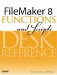# Div()

Category: Number

 Syntax: Div ( number; divisor )

Parameters:

numberAny expression that resolves to a numeric value.

divisorAny expression that resolves to a numeric value.

Data type returned: Number

Description:

Returns the quotient resulting from the division of the numerator number by the denominator divisor.

The Div() function is equivalent to Floor ( numerator / denominator ).

To obtain the remainder when a numerator is divided by denominator, use the Mod function.

Examples:

Function

Results

Div ( 30; 4 )

Returns 7 because 30/4 is 7, remainder 2.

Div ( 51; 8 )

Returns 6 because 50/8 is 6, remainder 3.FileMaker 8 Functions and Scripts Desk Reference
ISBN: 0789735113
EAN: 2147483647
Year: 2004
Pages: 352